Next: References Up: The spectrum of primordial Previous: The CDM spectrum.

## N-body simulations

A complete description of the processes of formation and evolution of cosmic structures is very complicated and far from having reached a satisfactory level of explanation. Formation of galaxies and galaxy clusters involves both gravitational dynamics and hydrodynamical processes. The situation is even less clear when trying to account for dissipative hydrodynamical effects. This really represents a serious limitation to our understanding of the large-scale galaxy distribution, since the observational mapping of the Universe mostly passes through the detection of luminous structures, i.e. of the regions where dissipation plays a fundamental role. A complete description of the attempts devoted to describing the hydrodynamical effects involved in galaxy formation is beyond the scope of these lectures.

In the framework of the gravitational instability picture, any non-gravitational effect is expected to be relevant only at quite small scales, where the characteristic time-scale for gravitational collapse,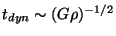, becomes comparable to the cooling time-scale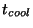. The determination ofis surely less reliable than that of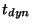, since it relies on the knowledge of cooling mechanisms, local chemical composition, etc. Nevertheless, it is reasonable to assume that at scales larger than that of a typical galaxy the dynamics is entirely determined by the non-dissipative gravitational interaction. At such scales, the description of the formation of large-scale structures is obtainable by solving the equations () for the evolution of the density inhomogeneities. However, the difficulty of analytically following the gravitational evolution when such equations are not linearizable forces one to resort to numerical methods. In this context, N-body simulations furnish a fundamental contribution towards understanding in more detail the nature of non-linear gravitational dynamics. In fact, N-body codes describe the evolution of non-linear gravitational clustering by following particle trajectories under the action of the gravitational force. Initial conditions (i.e., initial fluctuation and velocity fields) are fixed in a consistent way at a sufficiently early time, so that linear theory is a good approximation at all the relevant scales. Then, the final result of gravitational clustering is compared with the observational data, in order to assess the reliability of the initial condition model. It is however clear that, since small scale virialized structures probably have almost no memory of initial conditions, structures on larger scales (e.g., filaments or voids) are much more useful in giving constraints about the nature of the primordial fluctuations.

A basic parameter which measures the capability of N-body codes to faithfully represent gravitational clustering is the width of their dynamical range for mass and length resolution. Mass resolution is fixed by the total number of particles employed. Since a given mass is assigned to each particle, we should require at the linear stage that fluctuations on a mass scale below that of a particle were negligible. The dynamical range for length resolution is fixed by the ratio of the size of the simulation box to the softening scale for the computation of the gravitational force. Very detailed tests are always required to measure the resolution of numerical codes, in order to be sure about the reliability of the subsequent clustering representation.

Because of the limits imposed by computational costs and computer memory, different strategies can be adopted in order to compromise between between numerical resource and extension of the dynamical range. Accordingly, three main categories of N-body simulations can be devised, which essentially differ in their prescriptions for evaluating the gravitational force between particles.

(a)
Direct integration of the force acting on each particle, due to the presence of all the other particles . Within this approach, the force softening scale is usually very small and the particle trajectories are calculated with great precision. However, the price to be payed for this accuracy is the high computational cost, which goes like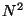(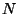being the number of particles). Therefore, only a rather limited number of particles (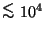) is usually employed.
(b)
Evaluation of the gravitational force by means of the particle-mesh" (PM) method, in which Poisson's equation is solved by a mass assignment to a discrete grid . This method is better suited when a large number of particles (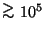) is required, although the small-scale resolution is limited by the grid spacing. For this reason, PM codes fail to describe in detail the structure of small-scale virialized clumps, although they are adequate to follow the evolution at intermediate and large scales.
(c)
Combination of the two above methods, in order to improve the force resolution of the PM code. The resulting particle-particle-particle mesh" (P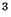M) code corrects the small scale force acting on each particle by summing over the contributions from neighbor particles . In this Chapter we will use the PM code written by Dr. R.Valdarnini to follow the development of non-linear clustering. A detailed technical description can be found in refs.[16,9].

For further technical details about N-body codes we refer to the standard textbook by Hockney & Eastwood , while we refer to the web-page of the Virgo consortium  for a description of the status-of-art cosmological simulations, ranging over a wide scale range.Next: References Up: The spectrum of primordial Previous: The CDM spectrum.
Waleska Aldana Segura 2001-01-16